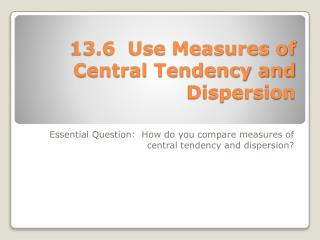Download Presentation13.6 Use Measures of Central Tendency and Dispersion13.6 Use Measures of Central Tendency and Dispersion - PowerPoint PPT Presentation

Download Presentation13.6 Use Measures of Central Tendency and Dispersion
An Image/Link below is provided (as is) to download presentation

Download Policy: Content on the Website is provided to you AS IS for your information and personal use and may not be sold / licensed / shared on other websites without getting consent from its author. While downloading, if for some reason you are not able to download a presentation, the publisher may have deleted the file from their server.

- - - - - - - - - - - - - - - - - - - - - - - - - - - E N D - - - - - - - - - - - - - - - - - - - - - - - - - - -
Presentation Transcript

1. 13.6 Use Measures of Central Tendency and Dispersion Essential Question: How do you compare measures of central tendency and dispersion?

2. 1. Order from least to greatest: 10.14, 11.2, 10.1, 10.08, 11.21 2. Jenna scored the following points in her last five basketball games: 28, 18, 24, 22, and 18. What is Jenna’s average score for the 5 games? 22 points 10.08, 10.1, 10.14, 11.2, 11.21 ANSWER ANSWER Lesson 13.6, For use with pages 875-878 Warm-up Exercises

3. Descriptions the Middle • Mean = average = x • x = x1 + x2 + … … + xn n • Median = the middle when the data set is ordered • Odd pieces of data  single number • Even pieces of data  mean of middle two numbers • Mode = most common data • Can be one mode, no mode, or multiple modes Measures of Central Tendency

4. Descriptions of the Spread • Range = the difference between the greatest value and the least • Mean Absolute Deviation = the average variation of the data from the mean • M.A.D. = |x1 – x| + |x2 – x| + … … + |xn – x| n Measures of Dispersion

5. 1000 + 1000 + 1181 + 1191 + 1200 + 1268 + 1328 + 2584 8 = 10,752 = x 8 EXAMPLE 1 Compare measures of central tendency The heights (in feet) of 8 waterfalls in the state of Washington are listed below. Which measure of central tendency best represents the data? 1000, 1000, 1181, 1191, 1200, 1268, 1328, 2584 SOLUTION = 1344

6. ANSWER The median best represents the data. The mode is significantly less than most of the data, and the mean is significantly greater than most of the data. EXAMPLE 1 Compare measures of central tendency The median is the mean of the two middle values,1191and1200, or1195.5. The mode is 1000.

7. 1000 + 1000 + 1181 + 1191 + 1200 + 1268 + 1328 = 7 8168 = x 7 for Example 1 GUIDED PRACTICE 1. What If ?In Example 1, suppose you eliminate the greatest data value, 2584. Which measure of central tendency best represents the remaining data? Explain your reasoning. SOLUTION = 1166 The median is 1191 The mode is 1000.

8. ANSWER The median best represents the data. The mode is significantly less than most of the data, and the mean is lower than most of the data. for Example 1 GUIDED PRACTICE

9. RUNNING EXAMPLE 2 Compare measures of dispersion The top 10 finishing times (in seconds) for runners in two men’s races are given. The times in a 100 meter dash are in set A, and the times in a 200 meter dash are in set B. Compare the spread of the data for the two sets using (a) the range and (b) the mean absolute deviation. A: 10.62, 10.94, 10.94, 10.98, 11.05, 11.13, 11.15, 11.28, 11.29, 11.32

10. ANSWER The range of set Bis greater than the range of set A. So, the data in Bcover a wider interval than the data in A. EXAMPLE 2 Compare measures of dispersion B: 21.37, 21.40, 22.23, 22.23, 22.34, 22.34, 22.36, 22.60, 22.66, 22.73 SOLUTION a. A: 11.32 – 10.62 = 0.7 B: 22.73 –21.37 = 1.36

11. | 21.37 – 22.226 | + | 21.40 – 22.226 | + … + | 22.73 – 22.226 | 10 | 10.62 – 11.07 | + | 10.94 – 11.07 | + … + | 11.32 – 11.07 | 10 EXAMPLE 2 Compare measures of dispersion b. The mean of setAis11.07, so the mean absolute deviation is: = 0.164 The mean of setBis22.226, so the mean absolute deviation is: = 0.3364

12. ANSWER The mean absolute deviation of set Bis greater, so the average variation from the mean is greater for the data in B than for the data in A. EXAMPLE 2 Compare measures of dispersion

13. RUNNING 2. for Example 2 GUIDED PRACTICE The top 10 finishing times (in seconds) for runners in a men’s 400 meter dash are 46.89, 47.65, 48.15, 49.05, 49.19, 49.50, 49.68, 51.09, 53.31, and 53.68. Compare the spread of the data with that of set Ain Example 2 using (a) the range and (b) the mean absolute deviation. a. A: 11.32 – 10.62 = 0.7 400 meter – 53.68 – 46.89 = 6.79 The range of finishing time for the men’s 400m dash (6.79) is greater than the range for set A(0.7)

14. | 46.89 – 49.819 | + | 47.65 – 49.819| + | 48.15 – 49.819| +…+ | 53.68 – 49.819| | 10.62 – 11.07 | + | 10.94 – 11.07 | + … + | 11.32 – 11.07 | 10 10 for Example 2 GUIDED PRACTICE b. The mean of setAis11.07, so the mean absolute deviation is: = 0.164 The mean of 400m men’sis 49.819, so the mean absolute deviation is : = 1.7246

15. How do you compare measures of central tendency and dispersion? Essential Question:

16. Textbook p. 877-878 Independent Practice